Softimage - Motion Builder Pipeline Tutorial By Ricardo Tobon Introduction This tutorial explains a simple skeletal hierarchy that can be used in a pipeline that uses Softimage for the rigging process and Motion Builder for the motion capture and animation sections of the project. To follow along and try out the concepts of this tutorial please download the Soft_Mobu.zip files. Softimage Skeletal Chains Softimage skeletal hierarchies are unique in the sense that every drawn chain is composed of 3 main elements: the root, the bones and the effector. Basically every skeletal chain is both an IK/FK chain (Figure 01).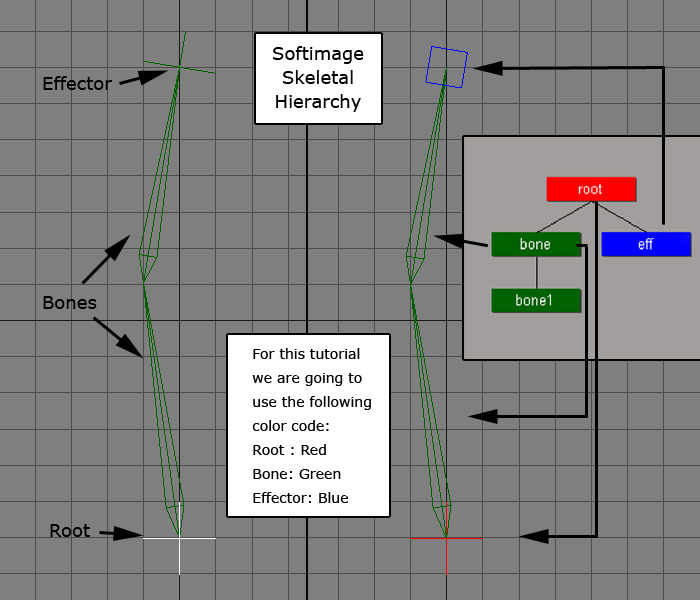Figure 01. The Rig Since there are 3 different elements to keep track for a skeletal hierarchy we are going to break the rig into the following sections: spine, neck, head, shoulders, arms, forearm twists, hands, fingers, legs and feet (Figure 02).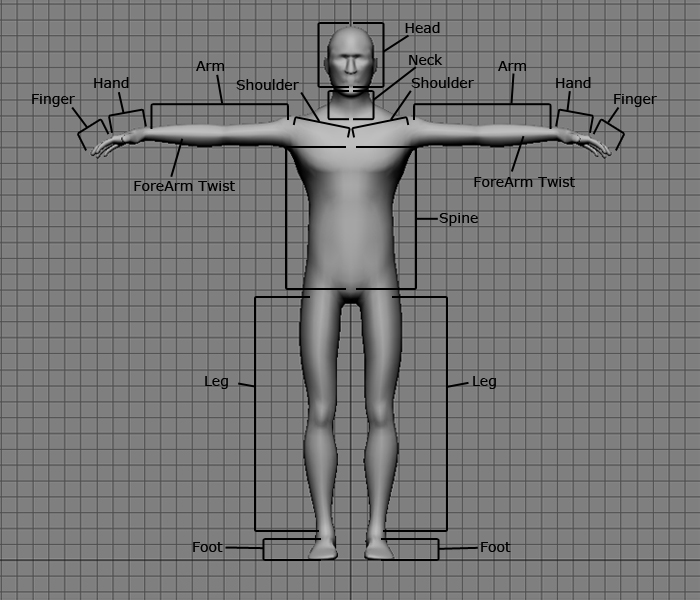Figure 02. Create the different skeletal hierarchies by selecting “Draw 2D Chain” or “Draw 3D Chain” from the “Create” section of the “Animate” toolbar and then clicking where you want to place the different bones, right click to finalize the operation (Figure 03).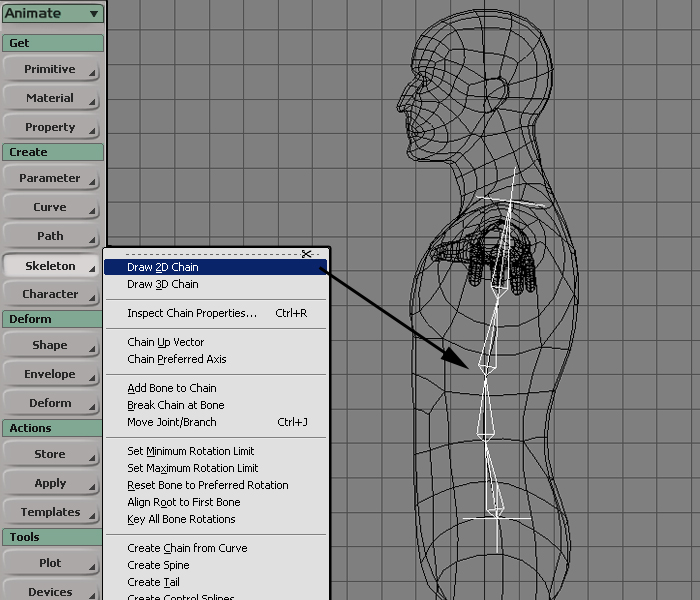Figure 03. Create a separate hierarchies for each previously mentioned section of the body and name the different elements like fig x illustrates (Figure 04 to Figure )Figure 04.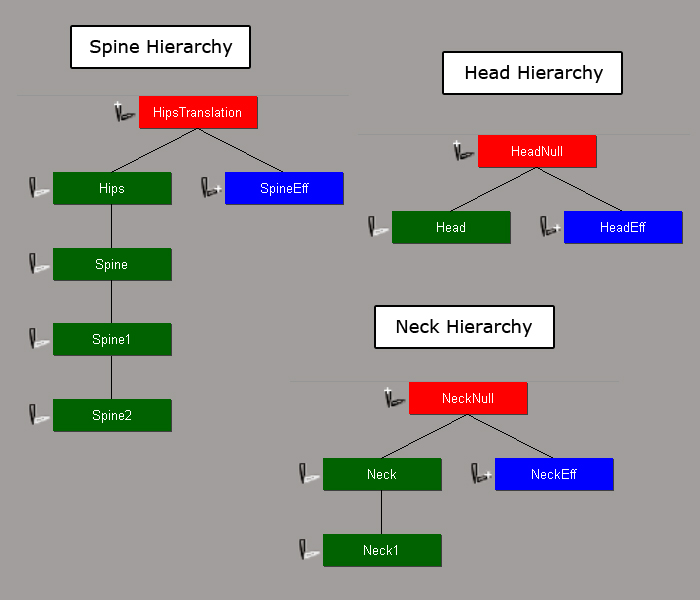Figure 05.Figure 06.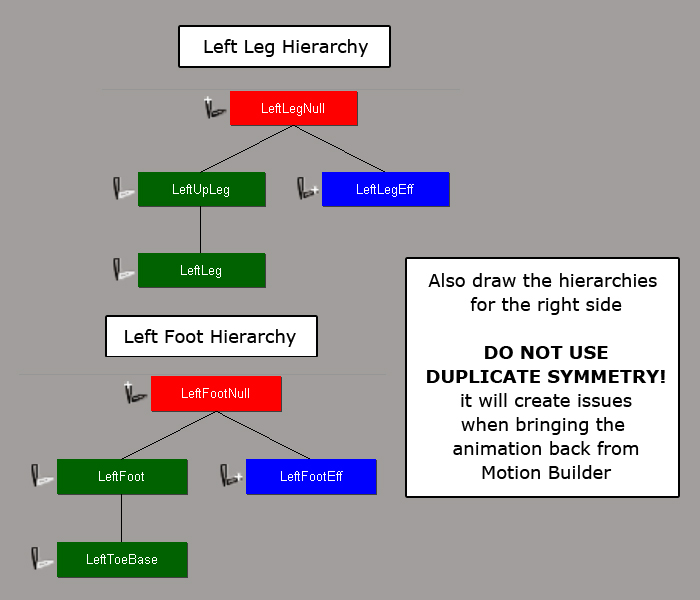Figure 07.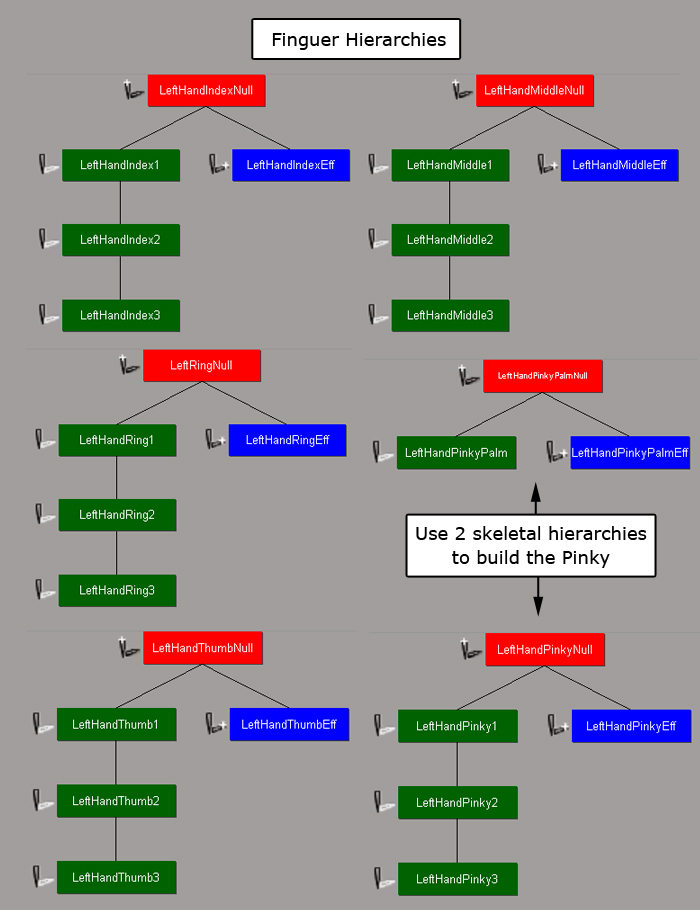Figure 08. Back Next Tutorials Main